Related Articles
Construction of a Quadrilateral
• Last Updated : 21 Feb, 2021

It is famously said that Geometry is the knowledge that appears to be produced by human beings, yet whose meaning is totally independent of them. Practical geometry is an important branch of geometry that helps us to study the size, positions, shapes as well as dimensions of objects and draw them with available geometrical devices. Geometry helps us in deciding what materials to use, what design to make and also plays a vital role in the construction process itself. Different houses and buildings are built in different geometric shapes to give a new look as well as to provide proper ventilation inside the house. In simple words, Geometry is a branch of mathematics that studies the sizes, shapes, positions angles, and dimensions of things. Flat shapes like squares, circles, and triangles are a part of flat geometry and are called 2D shapes. These shapes have only 2 dimensions, the length and the width. To determine geometric designs four important tools of geometry are-compass, straightedge, protractor, and ruler are usually used.

• Compass- helps to draw the different angles.
• Straightedge – it has two set squares, used to draw symmetric lines.
• Protractor – for determining and drawing correct and accurate angles.
• Ruler – Used to measure and draw lines to construct the figures.

### Quadrilateral

A quadrilateral is a closed polygon in geometry with four edges and four vertices. Other names for quadrilateral include quadrangle, tetragon, and 4-gon. In other words, any four-sided figure is referred to as quadrilateral. We shall learn in this article some different types of quadrilateral and how they are constructed using simple geometric tools. The word Quad means four and lateral means sides of the quadrilateral.  Hence, all closed figures with four sides are called quadrilaterals. The sides of any quadrilateral may be equal, unequal, parallel, or irregular which forms the basis of varying shapes in these four-sided shapes. Whichever is the shape, every quadrilateral has four sides, four vertices, and with all its angles adding up to be 360°. It is valid for all quadrilaterals.

The most common types of quadrilaterals are Squares and Rectangles. We have been studying these basic quadrilaterals since our primary classes, but in this part of the chapter we shall bring into light various other types of the quadrilateral, which show properties like square or rectangle yet are not named so. There are many different types of quadrilaterals among which some of them will be shown here.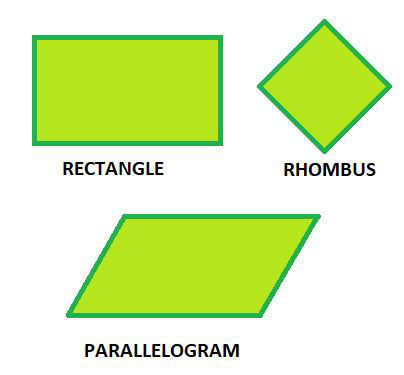The following types of quadrilateral will be constructed:

• If four sides and one diagonal of a quadrilateral are given.
• If two diagonals and three sides of a quadrilateral are given.
• If two adjacent sides and three angles of a quadrilateral are given.
• If three sides and two included angles of a quadrilateral are given.
• If other special properties of a quadrilateral are known.

Let us take up these constructions one-by-one.

### If four sides and one diagonal of a quadrilateral are given:

Follow the following steps to draw the quadrilateral:

Construct a quadrilateral PQRS where PQ= 5 cm, QR = 7 cm, RS = 6 cm, PS = 6.5 cm and PR = 8 cm.

Construct a rough sketch for reference first. Then follow the following: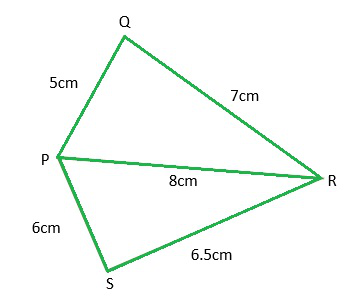Step 1: As we can see in the rough sketch, it is easy to see that ∆PQR can be constructed using SSS construction conditions. Draw ∆PQR.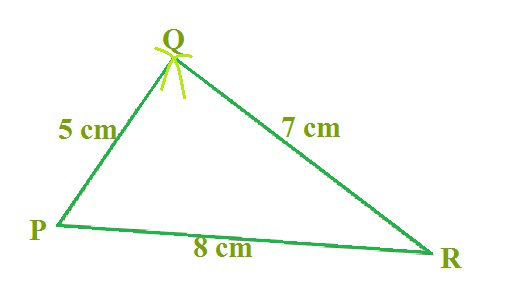Step 2: After this, we have to locate the fourth point S. This is on the side opposite to Q with reference to PR. For that, we have two measurements.

S is 6 cm away from point P. So, with P as center an arc is drawn of radius 6 cm.

Step 3: S is 6.5 cm away from R. So with R as center, draw an arc of radius 6.5cm

Step 4: S lies on the point of intersection of two arcs. Mark S and complete PQRS. PQRS is the required quadrilateral.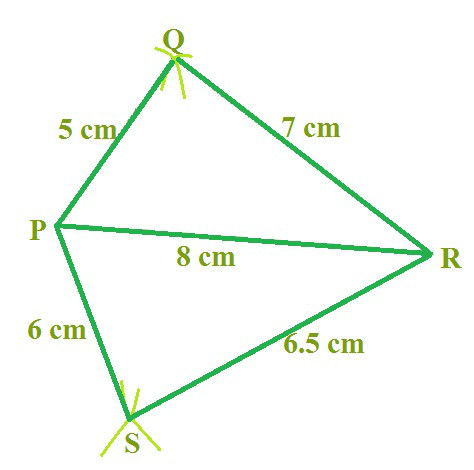Sample question: Construct a Quadrilateral ABCD with the following given

AB = 4.5 cm, BC = 5.5 cm, CD = 4 cm, AD = 6 cm, and AC = 7 cm

Answer:

We follow the above steps to make the quadrilateral ABCD with

AB = 5 cm, BC = 6 cm, CD = 6 cm, AD = 4 cm, AC = 7 cm

Hence, we get the final result as shown below,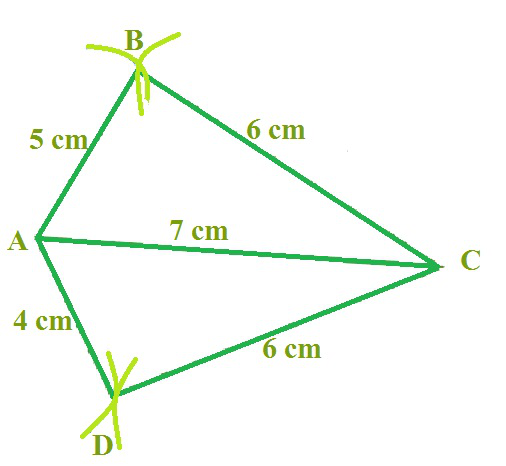Hence, ABCD is the required quadrilateral.

### If two diagonals and three sides of a quadrilateral are given:

Follow the following steps to draw the quadrilateral:

Construct a quadrilateral ABCD, given that BC = 5.5 cm, AD = 6.5 cm, CD = 5 cm the diagonal AC = 6 cm and diagonal BD = 8 cm.

First, draw a rough sketch of the quadrilateral ABCD.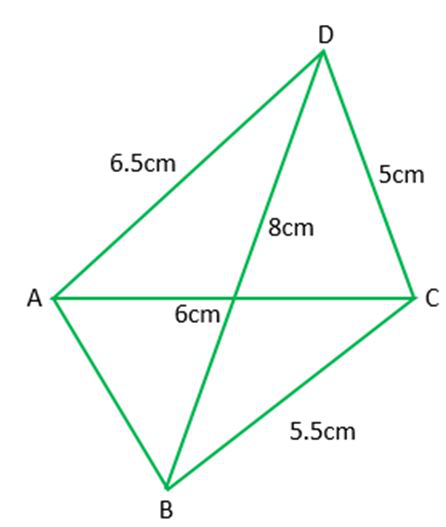Step 1: Draw ∆ACD using SSS construction.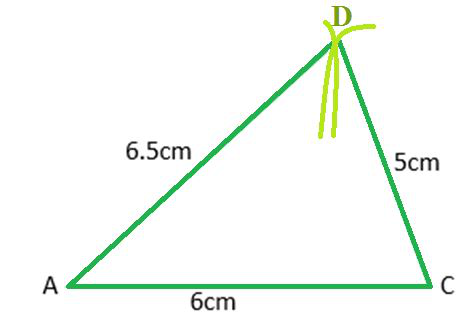Step 2: With D as center we draw an arc of radius 7 cm. With C as center we draw an arc of radius 4.5 cm.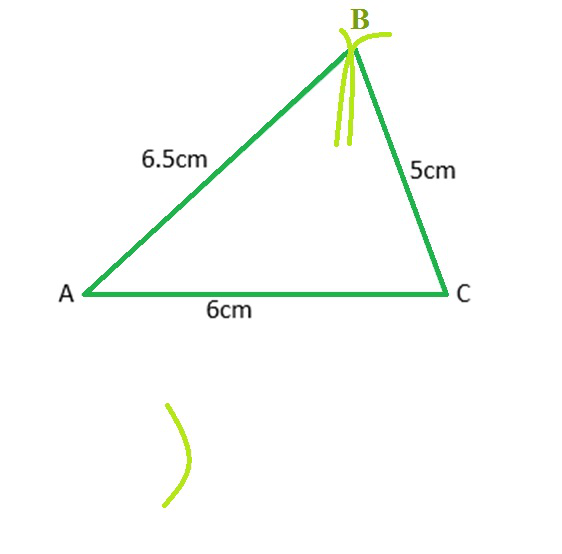Step 3: With C as centre we draw an arc of radius 4.5 cm.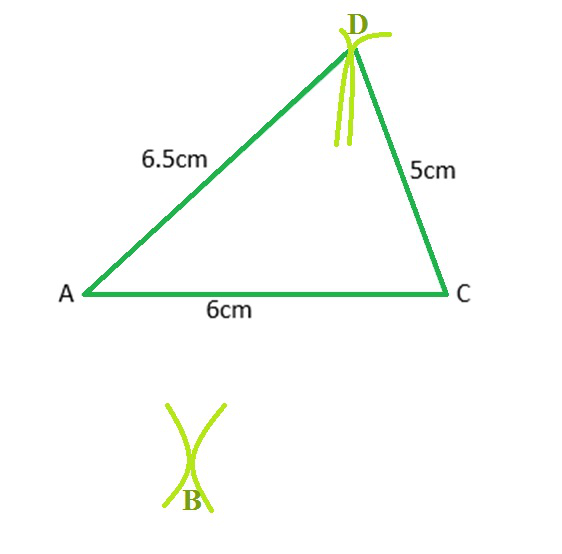Step 4: Since B lies on both the arcs, B is the point intersection of the two arcs. ABCD is the required quadrilateral.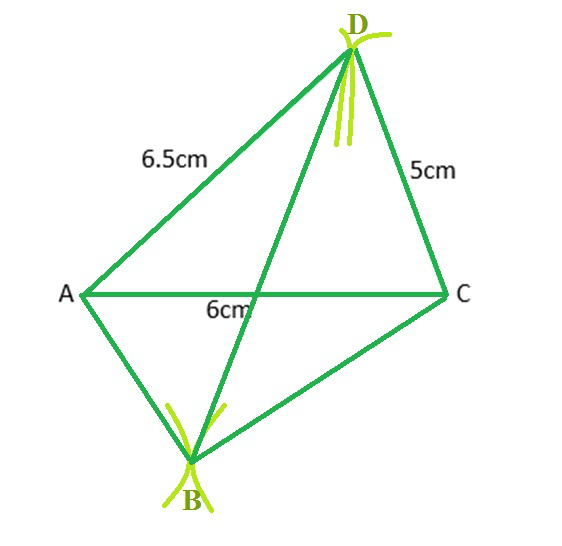Sample question: Construct a Quadrilateral GOLD with the following given

OL = 6.5 cm, GL = 7 cm, GD = 7 cm, LD = 6 cm, OD = 11 cm

Answer:

By following the similar steps as above, we can construct the quadrilateral GOLD with the following given

OL = 6.5 cm

GL = 7 cm

GD = 7.5 cm

LD = 6 cm

OD = 11 cm

Hence, the required quadrilateral GOLD.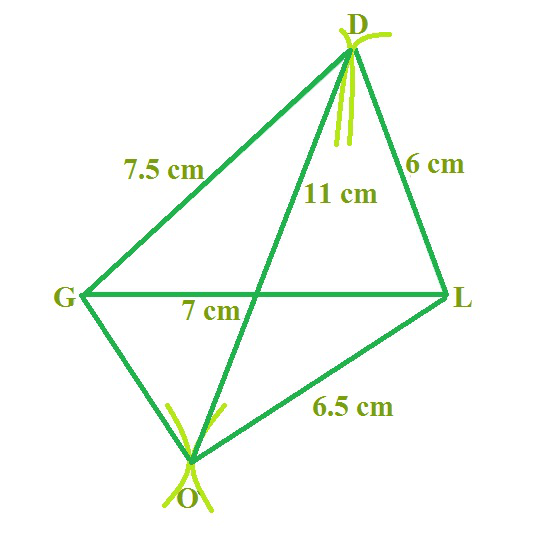### If two adjacent sides and three angles of a quadrilateral are given:

Follow the following steps to draw the quadrilateral:

Construct a quadrilateral MIST where MI = 3.5 cm, IS = 6.5 cm, ∠M = 75°, ∠I = 105° and ∠S = 120°.

Draw a rough sketch first.

Step 1: We first locate the points.

Step 2: Construct ∠IST = 120° at S

Step 3: Construct ∠IMT = 75° at M.

Step 4: We get the required quadrilateral MIST.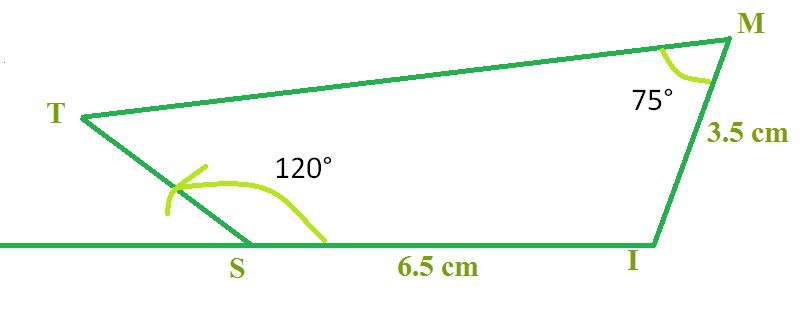Sample question: Construct a Quadrilateral PLAN with the given

PL = 4 cm, LA = 6.5 cm, ∠P = 90°, ∠A = 110°, and ∠N = 85°

Answer: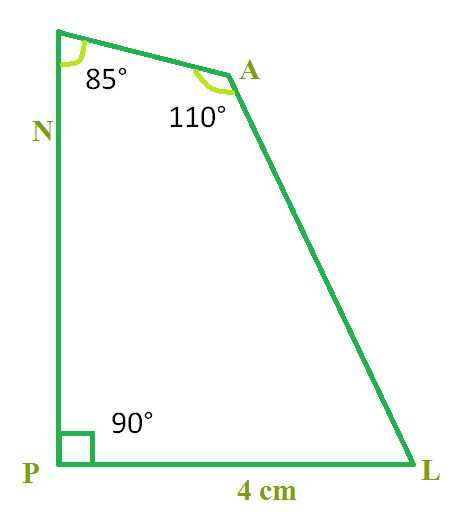### If three sides and two included angles of a quadrilateral are given:

Follow the following steps to draw the quadrilateral:

Construct a quadrilateral ABCD, where ST = 5 cm, TE = 6 cm, EP = 7.5 cm and ∠T = 105° and ∠E = 80°.

A rough sketch is drawn first

Step 1: Start with taking TE = 6 cm on Draw an angle of 105° along with TX.

Locate a point 5 cm away on this. We now have T, E, and S

Step 2: The fourth point P is on EY which is inclined at 80° to TE. So make ∠TEY = 80° at C on TE.

Step 3: D is at a distance of 7.5 cm on EY. With E as center, draw an arc of length 7.5 cm. It cuts EY at P.

Step 4: Complete the quadrilateral STEP.

Step 5: STEP is the required quadrilateral.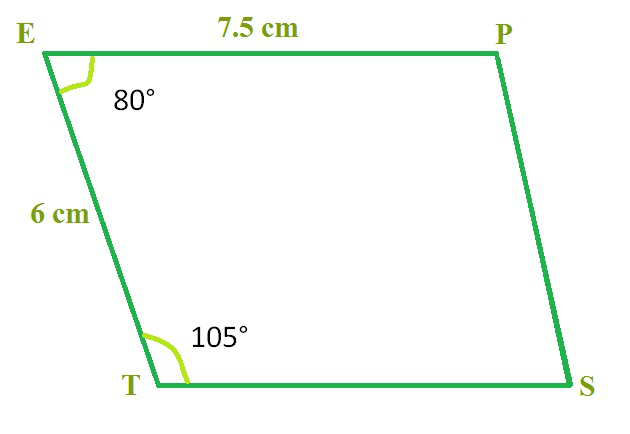### If other special properties of a quadrilateral are known:

Follow the following steps to draw the quadrilateral:

Question 1: Draw a square with side 6cm.

Answer:

We can see that only the side is given but we know that square is a special figure with all the angles equal to right angle. We can use this information and draw the square.

Step 1: Draw a rough figure.

Step 2: Taking 6cm as base draw a line AB.

Step 3: Draw right angles at vertex A and B.

Step 4: Cut 6cm on the rays drawn from A and B respectively.

Step 5: Name the cut points C and D respectively.

Step 6: Join CD.

Step 7: ABCD is the required square.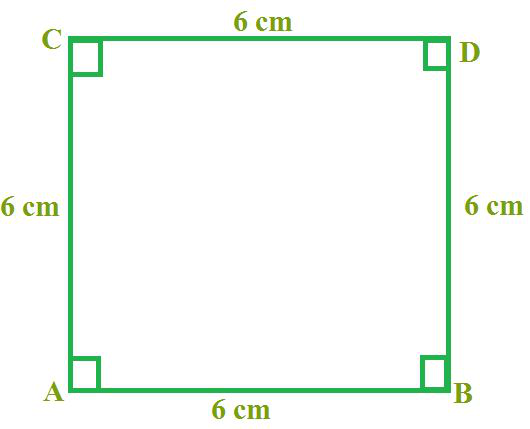Question 2: Construct a rhombus ABCD where AC = 6 cm and BD = 7 cm.

Answer:

The measurement of two diagonals is given. As we know,

The diagonals of a rhombus are perpendicular bisectors of each other.

Step 1: Firstly we will draw AC= 6cm and then we will draw its perpendicular bisector.

Step 2: Let them meet at 0.

Step 3: Cut off 3 cm lengths on either side of the drawn Bisector.

Step 4: We get B and D.

Step 5: Join the lines and we get the required rhombus ABCD.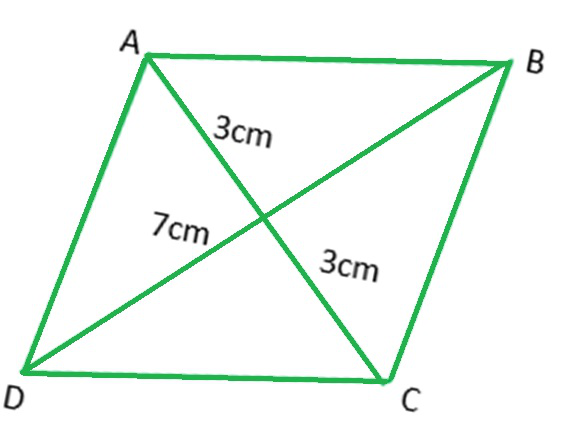Attention reader! Don’t stop learning now. Get hold of all the important DSA concepts with the DSA Self Paced Course at a student-friendly price and become industry ready.

My Personal Notes arrow_drop_up